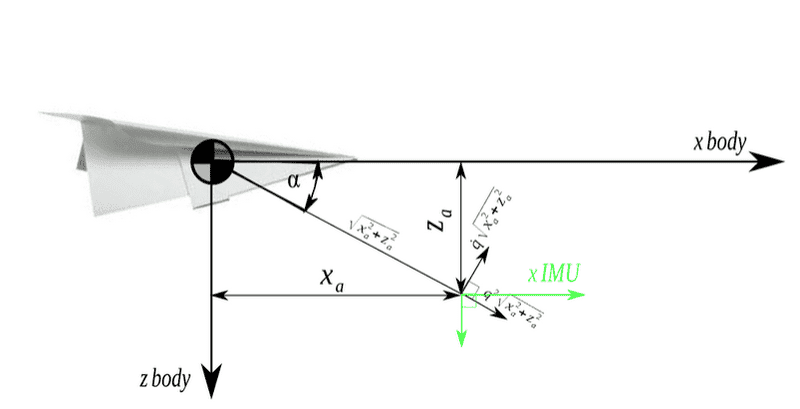# Calculating acceleration offset by CG

I'm trying to calculate the acceleration an accelerometer would read NOT placed in the Center of Gravity of the object. Let's look at the figure below. The accelerometer was placed in location (Xa,Za) and not in the CG of the object. Given that the accel's output is a_x and a_z at this location (Xa,Za)...what would've the accelerometers have read if placed on the C.G. of object?Here is an attempt at the solution (http://basicairdata.blogspot.com/2014/05/inertial-measurement-unit-placement.html) however I don't fully understand how they got the transformation matrix.

How does q_dot come into play in the calculations and how does alpha (angle) disappear in the matrix?

Last edited:

haruspex
Science Advisor
Homework Helper
Gold Member
2020 Award